MORE IN Structural Analysis 2
VTU Civil Engineering (Semester 5)
Structural Analysis 2
June 2014
Total marks: --
Total time: --
INSTRUCTIONS
(1) Assume appropriate data and state your reasons
(2) Marks are given to the right of every question
(3) Draw neat diagrams wherever necessary

1 (a) A series of wheel loads crosses over a girder of span 15m from left to right with 40 kN load leading as shown in Fig Q1(a). Determine i) Maximum B.M and ii) Maximum S.F at a section 4m from left support.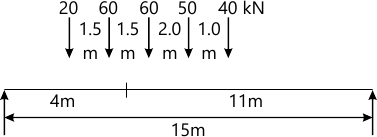10 M
1 (b) Draw ILD for S.F and B.M a section 3m from left support for a simply supported beam 12m long. Use the diagram to calculate maximum S.F and B.M at this section due to rolling load 5m long and 2kN/m intensity.
10 M

2 Analyze the frame shown in Fig. Q2, by slope deflection method and draw B.M.D.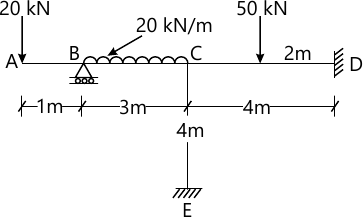20 M

3 A horizontal beam ABCD is carried on hinged supports and is continuous over three equal spans each of 3m. All the supports are initially at the same level. The beam is loaded as shown. Plot R.M.D> for the beam if the support 'A' settlers by 10mm, 'B' settlers by 30mm and 'C' settlers by 20mm. Use M.D. method. Take E=2.0×105 N/mm2, I=2.4×106 mm4.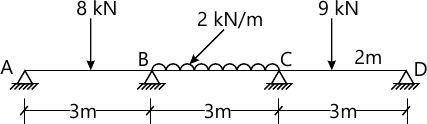20 M

4 Analyze the portal frame loaded as shown in Fig. Q4 and draw B.M.D. Use Moment distribution method.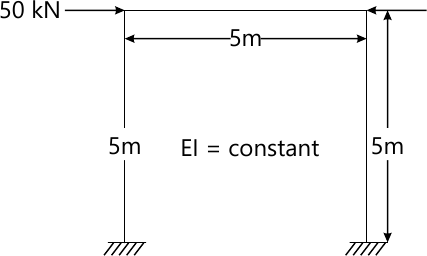20 M

5 Analyze the continuous beam shown in fig. Q5 by Kani's method. Draw S.F.D and B.M.D.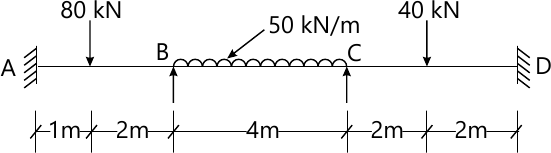20 M

6 Analyze the frame shown in fig Q6 by Flexibility method and draw B.M.D. Use system approach.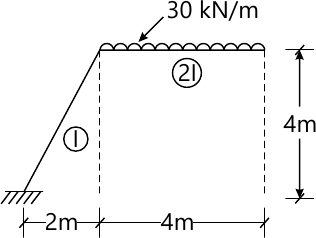20 M

7 Analyze the bent shown in fig. Q7 by stiffness method and draw B.M.D. Use system approach.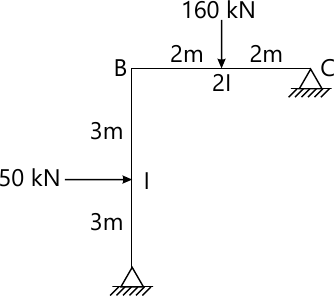20 M

8 (a) Explain: i) Free Vibration ii) Forced Vibration iii) Natural frequency iv) Degree of freedom v) Damping vi) Periodic motion.
12 M
8 (b) Find the natural frequency of the system. The mass of the beam is negligible in comparison to the suspended mass E=2.1×105 N/mm2 and I=28.125×106 mm4 for beam.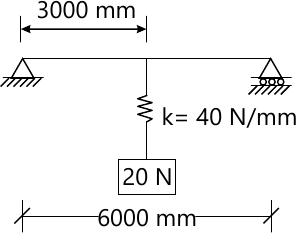8 M

More question papers from Structural Analysis 2